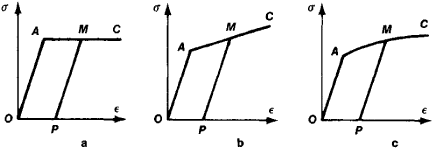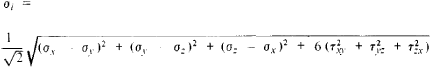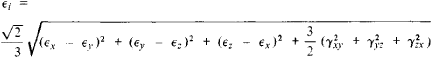# Plasticity, Theory of

## Plasticity, Theory of

the branch of mechanics that investigates the deformation of solids beyond the elastic limits. Not directly concerned with the physical explanation of the properties of plasticity, it studies the macroscopic properties of plastic solids and deals with methods for the determination of the distribution of stresses and deformations in solids that undergo plastic deformation.Figure 1. Stress-strain (σ-∊) diagram for a specimen of soft low-carbon steel: (OA)elastic deformation, (A) elastic limit (more precisely, limit of proportionality), (B) yield point, (BC) yield region, (MP)unloading line

To determine the plastic properties of metals, experiments are performed on the tension and compression of flat or cylindrical specimens and the deformation of thin-walled cylindrical tubes under the action of tensile force, torque, and internal pressure— that is, experiments permitting independent recording of forces and deformations. The deformation of a given material is characterized by a stress-strain diagram (Figure 1). The theory of plasticity uses various hypotheses to idealize the behavior of real materials during plastic deformation. The theory usually approximates the stress-strain curve by the two-part scheme illustrated in Figure 2: the straight line segment OA corresponds to the elastic state of the material, and the segment AC corresponds to the state of plasticity.Figure 2. Idealized representations of the stress-strain (σ-∊) relationship: (a) ideally plastic material, (b) material with linear hardening, (c) material with nonlinear hardening

The stress and strain states of a material during plastic deformation depend essentially on the loading history. Thus, reloading of a specimen after its unloading (line PM, Figure 1) raises the elastic limit of the material (point M instead of point A); this is called work hardening. Various plastic deformations may therefore correspond to a given stress, depending on the sequence of strain states by which it was achieved. The setting up of a model of a plastic solid consists in determining the relationship between the tensors defining the complex stress and strain states of materials.

The theory of small elastico-plastic deformations (deformation theory) has been widely accepted. It formulates the relationships between the values of the stressesand the values of the strains at the same pointwhere σx, σy, and σz are the normal stresses in the coordinate planes at the given point; τxy, τyz, and τzx are the shearing stresses; ∊x, ∊y, and ∊z. are the tensile strains; and γxy γyz, and γzx are the shear strains. For the case in which the values of the deformations at a given point increase, the quantities σi, and ∊i are assumed to be interrelated independently of the type of the strain state. The deformation theory of plasticity is applicable, strictly speaking, only in cases of simple loading, when all of the components of the strain state increase proportionally to a single parameter.

More general is the flow theory, which links strain and stress increments with the stress components.

The theory of plasticity plays a large role in engineering. For example, it is closely connected with important problems in structural design and with the investigation of industrial processes involving the plastic deformation of metals. Important applications of the theory of plasticity also concern the theory of stability of plates and shells.

### REFERENCES

Il’iushin, A. A. Plastichnost’, osnovy obshchei matematicheskoi teorii. Moscow, 1963.
Ishlinskii, A. Iu. “Plastichnost’.” In Mekhanika ν SSSR za 30 let. Moscow-Leningrad, 1950.
Kachanov, L. M. Osnovy teorii plastichnosti. Moscow, 1956.
Nádai, A. Plastichnost’i razrushenie tverdykh tel. Moscow, 1954. (Translated from English.)
Prager, W., and P. G. Hodge. Teoriia ideal’no plasticheskikh tel. Moscow, 1956. (Translated from English.)

A. S. VOL’MIR

Site: Follow: Share:
Open / Close